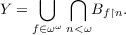#### Vol. 97, No. 1, 1981

 Recent Issues Vol. 325: 1 Vol. 324: 1  2 Vol. 323: 1  2 Vol. 322: 1  2 Vol. 321: 1  2 Vol. 320: 1  2 Vol. 319: 1  2 Vol. 318: 1  2Online Archive Volume: Issue:The Journal Subscriptions Editorial Board Officers Contacts Submission Guidelines Submission Form Policies for Authors ISSN: 1945-5844 (e-only) ISSN: 0030-8730 (print) Special Issues Author Index To Appear Other MSP Journals
Generic Souslin sets

### Arnold William Miller

Vol. 97 (1981), No. 1, 171–181
##### Abstract

sforcing we create generic Souslin sets, which we use to answer questions of Ulam, Hansell, and Mauldin. For X a topological space a set Y X is analytic in X(also called Souslin in X or Σ11 in X) iff there are Borel sets Bs for s ω such that:For X = 2ω (the Cantor space) a set Y X is analytic iff it is the projection of a Borel subset of 2ω × 2ω. Given R P(X) (the power set of X) let B(R) be the smallest family of subsets of X including R and closed under countable union and complementation (i.e., the σ-algebra generated by R). If X is a topological space and R the family of open sets then B(R) is the family of Borel subsets of X. The following question was raised by Ulam.

1. Does there exist R P(2ω) such that R is countable and every analytic set in 2ω is an element of B(R)?

Rothberger showed that assuming CH there is such a R. We will show that it is consistent with ZFC that there is no such R.

2. Does there exist a separable metric space X in which every subset is analytic but not every subset is Borel?

This was raised by R. W. Hansell. Clearly CH implies no such X exists. We show that it is consistent with ZFC that such a X exists.

Let R = {A × B : A,B 2ω}, the abstract rectangles in the plane. Let S(R) be the family of subsets of 2ω×2ω obtained by applying the Souslin operation to sets in B(R). The next question was asked by D. Mauldin.

3. Does S(R) = P(2ω × 2ω) imply B(R) = P(2ω × 2ω)?

We show that the answer to this question is no.

##### Mathematical Subject Classification 2000
Primary: 03E35
Secondary: 03E15, 54H05
##### Milestones